Machine Learning with Spreadsheets! Part 1: Gradient Descent and Backprop for Beginners

🎮 Skynet: Rise of the Machines 🎮

They say it got smart, a new order of intelligence. Then it saw people as a threat…decided our fate in a microsecond:
EXTERMINATION.
— Terminator (on Skynet)

I flipped through the first few pages and I was hooked. My mind was racing with possibility. I was going to be a superhero. Save lives. And my super power? Artificial intelligence.

The thought of building machines that could program themselves and “learn” had my grandiose Silicon Valley dreams running wild. AI had come a long way since Watson won Jeopardy! and now it can do things like detect cancer and drive cars.

Maybe it was all hype…

Or maybe I SHOULD be scared like Elon’s been saying

Either way, I was excited to jump on this fast-moving train and claim my stake in Silicon Valley’s modern day gold rush! I wasn’t going to be left behind.

By page 10, I was utterly lost.

Alphas, betas, etas….WHAT had I gotten myself into?!?! I did ok in high school calculus, but that was many years ago and I was no John Nash. I wanted to learn to build neural nets that could do some good in the world. Now I felt overwhelmed. The thought of trying to learn Greek math, Python code, and Tensorflow at the same time just seemed too daunting.

This used to be me. A finance guy by trade who had never typed a line of code in his life, but wanted to learn what all the machine learning hype was all about. One part scared, one part excited. Here’s what I learned though…

Machine learning can be SIMPLE and FUN

And once you understand just the basics, it does feel like you have this bad-ass superpower that would make even JARVIS jealous. By the end of this post, you will understand what it means for machines to “LEARN.” I’ll SHOW you the key principles of deep learning/neural nets in a simple, fun, visual way so you can throw on your cape and go save some lives. No coding experience or PhD needed.

Welcome to Excellerated Machine Learning

This series is written for people who want to get under the hood and take their learning to the next level. Whether you are taking Andrew Ng’s machine learning course on Coursera, pursuing a nanodegree on Udacity, or you just have a curious mind…this is for you.

Using spreadsheets (no code!), you’ll discover the step-by-step details behind how machines “learn”. Gradient descent, backprop, and partial derivatives…these are the building blocks of what makes up the “learning” in “machine learning.”

In part 2 of this series, I’ll walk through how machine see (CNNs — convolutional neural nets).

Sit back and enjoy the ride.

The Spreadsheet — Bringing sexy back? Or did it ever really leave???

Every day, it seems like there’s another cutting edge, distributed data blah-blah du-jour programming language being introduced while another one becomes a dinosaur …gone forever.

You know what has stood the test of time? Good ‘ol Mr. Excel.

I would argue the spreadsheet is the world’s most functional programming language around and it’s the best way for you to SEE the math behind how machines learn in a simple, step-by-step approach. Once you understand the principles of machine learning, you can focus on tackling the tools of the trade (libraries, Python code, etc.).

To have some fun while we learn, we’ll play a Skynet/terminator-themed spreadsheet game and each “level” in the game represents a key learning lesson on our way to understanding the entire process.

Before jumping into the game, let’s start with an analogy so we can see the big picture:

Imagine Skynet teleports a terminator to earth with the mission to find and terminate all of humanity…
The terminator lands at the top of Mount Errorest…it takes a look around, but doesn’t see any humans, so it wants to get to the bottom of the mountain.
So which way does it step? Down. It wants to DESCEND down the mountain’s GRADIENT (“slope”) and continue taking steps in the steepest direction.
After taking a step, it looks around again, and continues to take steps 1 at a time down the mountain until it reaches humans at the bottom.
Gradient descent is like terminator’s compass and it will always lead it down the mountain towards humans. It is the process used to tweak the model’s weights and improve the accuracy…after updating all the weights, the net effect is that you are lower on Mount Errorest (your model’s error is lower and your accuracy has improved).

Gradient Descent Game — Learning Levels

We’ll start with setting up the game (reviewing our model’s inputs and pre-processing the data).

GAME INSTRUCTIONS

SETTING UP THE GAME — TERMINATOR PLOT

Here’s the basic plot of the never-ending ‘Terminator’ movie series:

Disguised as a human, a cyborg assassin known as “The Terminator” (Arnold Schwarzenegger) travels from 2029 to 1984 to kill Sarah Connor. Sarah is targeted because Skynet, a super computer AI system, knows that her unborn son (John Connor) will ultimately lead the human resistance to victory in a bitter future war with the machines. Since Skynet saw people as a threat to its own survival, it made its’ mission to:
TERMINATE HUMANITY.

In each terminator movie, the humans survive, BUT…Skynet learns how to wire up a better terminator.

In our example, we’ll see how Skynet learns to predict whether any given terminator will terminate humanity (yes or no) using a neural network. This is a binary classification problem.

For Skynet to generate its’ prediction, we’ll feed the features of sample terminators into the model as inputs (each terminator model has varying degrees of strength, combat skills, and human likeness).

Here are the inputs:

• Strength: 0 (weak) to 1000 (super-human)
• Combat skills: 1 (couldn’t hurt a fly) to 4 (lethal)
• Human likeness: (the best terminators look human): robot (worst), Arnold-like (better), creepy cop (even better), Elon Musk-like (best)

In the training data, we also know whether or not that specific terminator model succeeded in ending mankind.

At first, Skynet has a 50/50 chance of predicting whether humans survive (yes or no). Over time though, it learns to improve its prediction accuracy so it can ultimately predict whether an army of new terminators will terminate all of mankind or not 😨.

TURNING EVERYTHING TO NUMBERS

Before Skynet can learn, we have to turn all inputs (like human_likeness) and outputs (yes/no) into numbers as computers need numbers to learn.

Categorical inputs: At first, you may think, let’s just call a robot a ‘1’, arnold a ‘2’, the creepy cop a ‘3’, and elon musk a ‘4’….

Well, we can’t actually do that because these aren’t linear — Arnold (2) isn’t 2x as human looking as the robot (1) and Elon Musk (4) isn’t 2x more human looking as Arnold (2). Instead, we split this ‘categorical’ input into 4 separate inputs:

1. robot? yes (1) or no (0)
2. arnold? yes (1) or no (0)
3. creepy_cop? yes (1) or no (0)
4. elon_musk? yes (1) or no (0)

For the output (humans_exterminated?), a yes will be labeled as ‘1’ and a no is a ‘0’. The technical term for this is ‘one-hot encoding’ or ‘creating dummy variables’.

Here’s our updated training data. We now have 6 inputs to feed our model:

GET THE OTHER INPUTS (STRENGTH AND COMBAT SKILLS) ON THE SAME SCALE

When we train, we don’t want to assume that the robot’s strength (800) is 200x more important than its’ combat_skills (4). Instead, we’ll get all features on the same scale and then let Skynet determine what carries the most weight in the prediction. This scaling step speeds up the learning process and gets everything on a level playing field.

To get the data on the same scale, we’ll use an approach called ‘standardization’.

This takes a bell-curve and centers the data around a mean of 0 for both the strength and the combat_skills. This means that we turn the average into a zero and then most (68%) of our data will be between a range of +/- 1 standard deviation and 95% of our data will be in a range of +/- 2 standard deviations.

If we were to standardize and plot strength, here’s what it would look like:

Now, let’s get under the hood a bit…

After we scale the strength and combat_skills, here’s our updated training data:

🎮 Now that our data is ready, it’s time to play Gradient Descent! 🎮

LEVEL 0: TERMINATOR — NETWORK OVERVIEW

The game is broken into key “learning levels” within 3 stages of the game:

1. The forward pass — FORWARD PROPAGATION — the input data moves through the network and the model generates a prediction (probability that humans are terminated: > 0.5 = humans terminated, < 0.5 = humans live)
2. Judgment Day — we calculate the error by comparing the prediction to the known training output
3. The backwards pass — BACKPROPAGATION — Skynet learns how much to tweak each weight in the network to improve its’ prediction accuracy during the next training run

In level 0, we’ll review the network architecture and see how the data moves through the network from left to right.

Level 0 — Network overview: 6 x 2 x 1 (3 layers)

• 6 inputs (strength, combat, robot, arnold, cop, elon)
• 2 hidden neurons (these hidden neurons each have an input and an output) — the hidden neurons allow us to learn complex patterns/relationships between the inputs that aren’t visible to the human eye.
• 1 output neuron (with an input and an output) — the output of the output neuron is our prediction (a number between 0 and 1). If the output is > 0.5, humans are terminated…< 0.5, humans live.

Weights/wiring:

• 12 weights between the inputs and hidden neurons — think of these as Skynet’s wiring. When Skynet trains, it can’t control the inputs, but it can control the wiring. To improve its’ prediction accuracy, it will make slight adjustments to these wires in each training run.
• 2 more weights between the hidden neurons and output neuron

In practice, there is no limit (other than processing power) to how many hidden layers you use or how many hidden neurons are in each hidden layer. This concept of adding more and more hidden layers between your inputs and outputs is where the term ‘deep learning’ comes from.

Note: the data set and network architecture was adapted from an example found on Udacity and it does not include bias terms on the hidden layers. A bias term is another weight that is ADDED to a neuron’s input.

In the next level, we’ll feed real numbers into the model and calculate the inputs of our hidden neurons.

LEVEL 1: SARAH CONNOR — NEURON INPUT

In our game, we’ll use 1 sample terminator from the training data and the inputs are already scaled.

The starting weights (the robot’s “wires”) were randomly chosen between (0.8) to 0.8, centered around 0.0. By randomly assigning initial weights, we ensure different signals (‘inputs’) are calculated for each of the 2 hidden neurons and the network learns faster.

The input into each hidden neuron is a linear sum of the inputs and the weights connecting the inputs to the hidden neuron.

For each hidden neuron, we take the 6 inputs, multiply each one by the “input (i) to hidden (j) weight” and then add up the 6 calculations. For the first hidden neuron, we use the h1 weights and for the second hidden neuron, we use the h2 weights.

In the next level, we’ll see how each hidden neuron’s input is fed into the neuron’s output function.

LEVEL 2: JOHN CONNOR — NEURON OUTPUT

The input and output functions of the neuron is why we have all these brain analogies. The input acts like an electric signal being passed to the output function and if this input is strong enough (value is high), the output function/neuron will “fire” (produce an output that is also a high value) and this signal is passed to the next neuron of the network.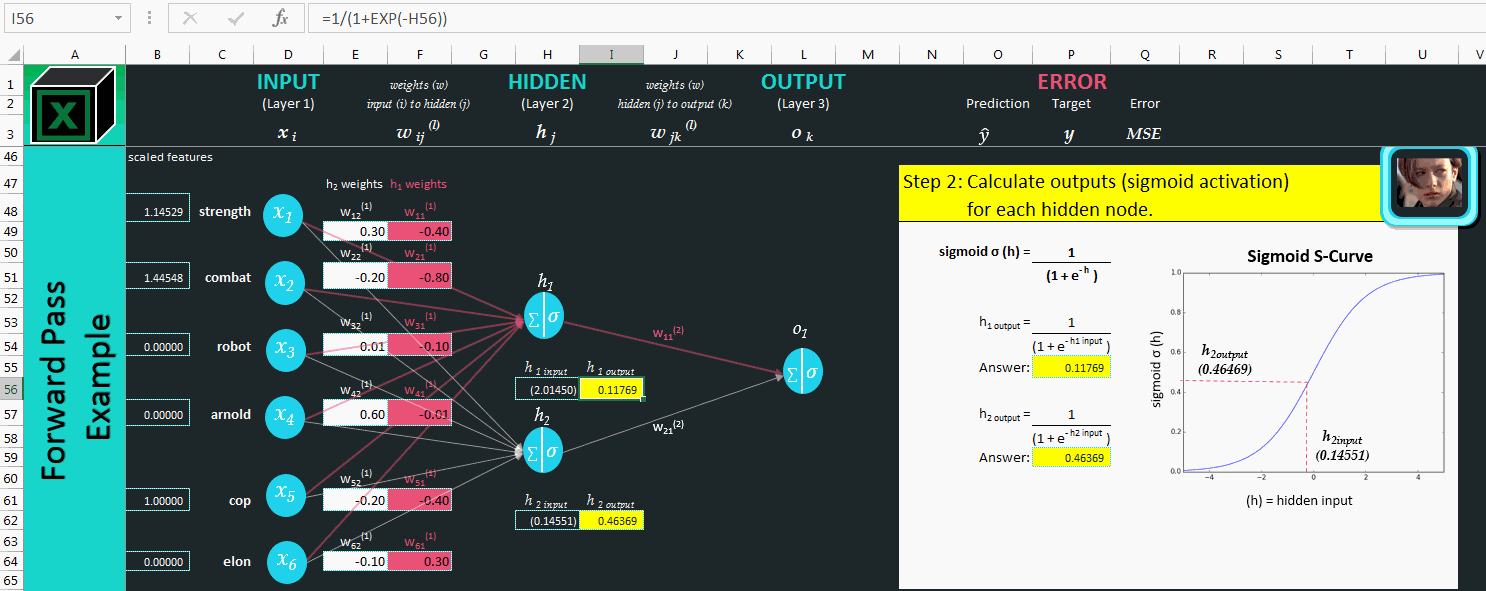Level 2: Sigmoid Activation Function Squashing the Hidden Inputs — ExcelwithML.com

The output function we’ll use, the sigmoid function (also called the logistic function), produces outputs in the range of 0 to 1.

The hidden input is fed into the function and the output function squashes it so the hidden output is a value between 0 and 1. This function is 1 way to introduce non-linearity (notice how the graph is an “S-curve” and not a straight line) into a neural network and you can think of it as a probability score. This non-linearity helps the model learn non-linear, complex patterns in input data that are often naked to the human eye. A high input produces an output near 1 and a low input produces an output near 0.

Since deep learning is simply deep pattern recognition, these non-linearity functions improve a model’s prediction accuracy.

Now that we‘ve calc’d the hidden outputs, we’ll calculate the input to our output neuron (o1) using another linear sum.

REPEATING LEVEL 1 — ANOTHER LINEAR SUM — NEURON INPUT

On to Skynet’s prediction…time to check the fate of mankind!

LEVEL 3: WILL HUMANS SURVIVE?

Skynet’s prediction function is another sigmoid (same formula as earlier) which squashes the output between 0–1. If we set the classification threshold at 0.5, then:

• If the value is > 0.5 — BYE, BYE HUMANS! 👀
• If the value is < 0.5 — HUMANS SURVIVE! 🙏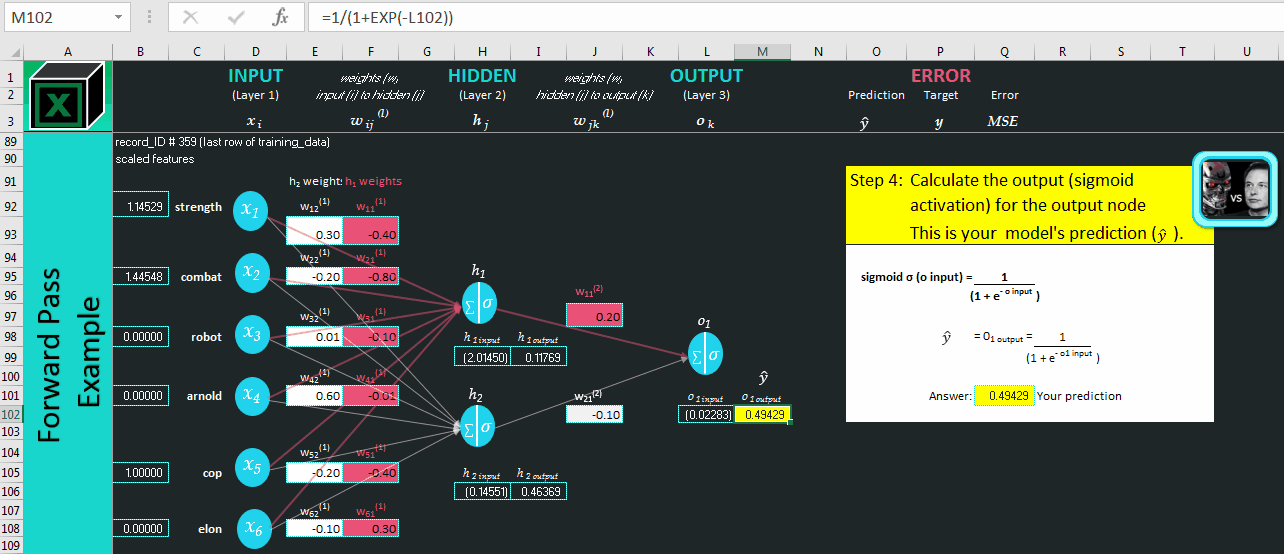Level 3: Skynet Calculating the Probability Humans Will Survive — ExcelwithML.com

Skynet’s prediction is 0.49429 or 49.429% chance that this terminator will terminate humans, but it’s basically a coin-flip. Since this is the first time Skynet is training, it’s not very confident in its’ prediction.

In the next level, we compare this prediction vs. the known/target outcome (1 or 0) from our training data to calculate the model’s error.

After we know the error, we‘ll complete the backwards pass to understand how to adjust each of our 14 weights to improve the prediction.

LEVEL 4: JUDGMENT DAY — CALCULATE ERROR

In the training data, the target is 0 (human survive) for this sample terminator so our error formula becomes:

`error_formula: = (target_outcome - model_prediction)`
`insert_values: = (0 — 0.049429)`
`answer: = (0.49429)`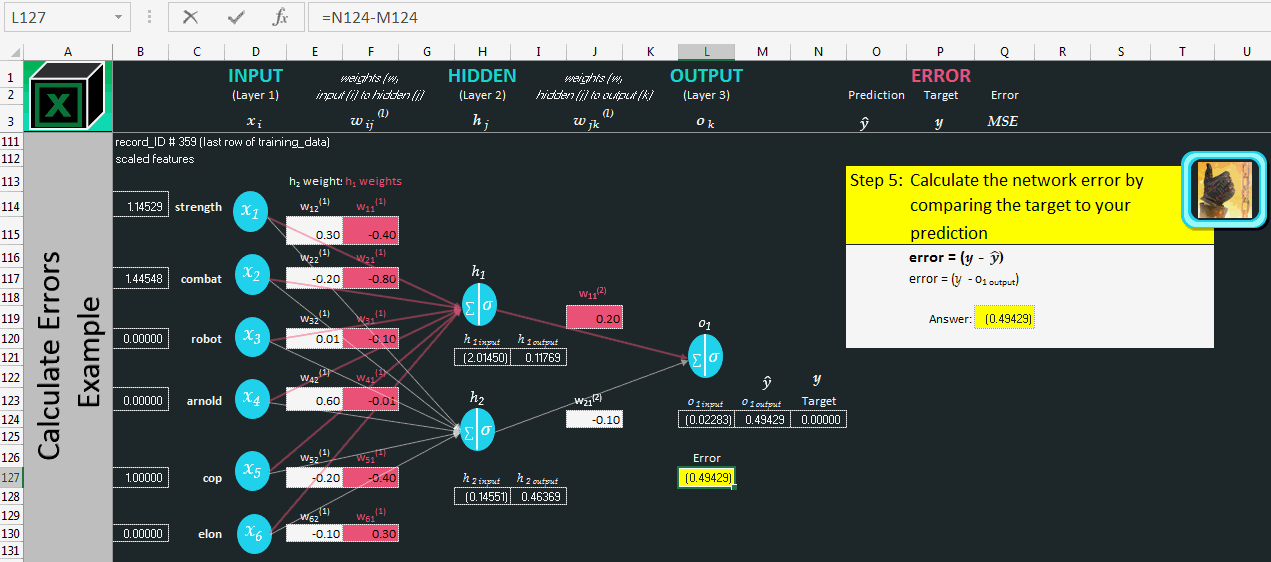Level 4: Judging Skynet’s Prediction vs. the Known Target Outcome — ExcelwithML.com

MSE (Mean Squared Error)

• Now that we have calculated the error (also called the ‘loss’), we need to square it in our next step to ensure we have a positive error. Why do we want a positive error? Since the goal of training is to minimize the error (get it as close as possible to 0), if we started out with a negative error (by not squaring it in our example), our model would be incentivized to calculate an error which ultimately went to negative infinity.
• We also need to average the squared errors across all sample terminators — the mean. Since this illustration only uses 1 training example, the mean squared error (MSE) is equal to the squared error.

In the MSE formula referenced in step 6 above, you will notice the formula has a (1/2) term at the front. This 1/2 term is ignored when you calculate the MSE. It is only used when we calculate the partial derivative of the total error function (level 6 below). When we calculate the derivative, the “squared 2” is multiplied times the (1/2) to equal 1. The 1/2 term simply makes the math easier.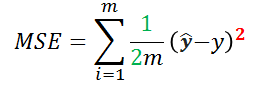This 2 is multiplied by the 1/2 term when we calculate the partial derivative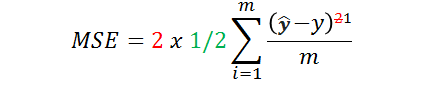The 1/2 term simplifies the math when we calculate the derivative in level 6 below

Now that we’ve calculated the MSE, it’s time to tweak the weights (all terminators use the same weights) to improve the prediction.

The backwards pass levels, or ‘backpropagation’, is where Skynet begins to learn.

The goal is to improve the prediction (currently at 0.49429) so it’s closer to the target outcome (0).

• Can the inputs change? NO
• Can the weights (terminator’s “wires”) change? YES!

To do this, we need to know which direction to move each weight to minimize our error. Backpropagation gives us the direction of the slope of the MSE (y-axis) with respect to the weight (x-axis).

This slope or “gradient” can be positive, negative, or flat and the weight will always be moved in the opposite direction as the gradient to decrease the error.

For now, take note of this rule and we’ll walk through the calc below…

This can be read multiple ways and they all mean the same thing:

`- The slope of the MSE (y-axis) with respect to the weight (x-axis)`
`- The partial derivative of the MSE with respect to weight 1`
`- The partial change of the MSE with respect to weight 1`
`- The MSE gradient with respect to weight 1`
`- Rise over run (a change in starting and ending point on y-axis divided by a change in starting and ending point on x-axis)`

Backpropagation vs. gradient descent — what’s the difference?

• Backpropagation (this level) is the process used to calculate the gradient of the MSE with respect each weight (see steps 7.1 to 7.8 below)
• Gradient descent (final level) is the process of slowly adjusting each weight to move in the opposite direction (“descent”) of the gradient

‘Chain rule’ in backpropagation:

To calculate the gradient of a weight with respect to the MSE we have to use the chain rule. The chain rule says that if we want to understand how 1 input (like a weight) affects a final output (like the MSE), we have to understand all of the intermediate changes along the way.

Each of these intermediate changes is a partial derivative (“change”) and the below steps illustrate the chain rule using 1 of the 2 ‘hidden to output weights’.

While the order of the multiplication doesn’t matter, it is more intuitive to start with the error (A) and work backwards like the Excel model below since this is BACKpropagation…we are propagating our error backwards.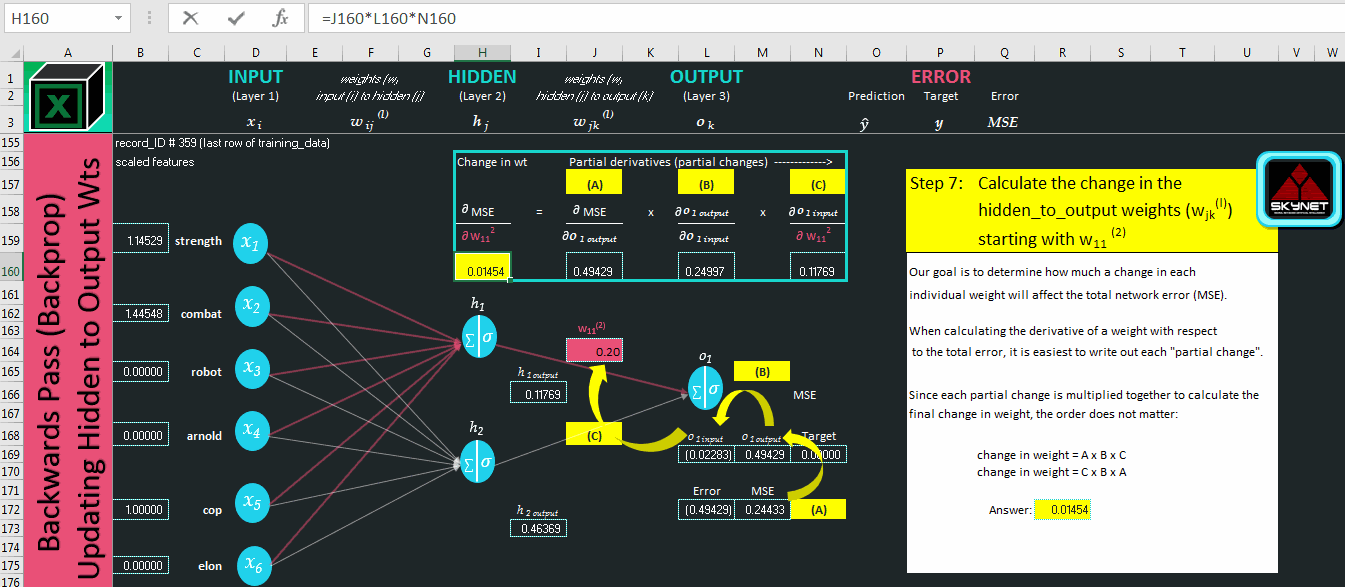Level 5: Backprop — Calculating how much a change in the “hidden to output” weight impacts the error — ExcelwithML.com

In levels 6, 7, and 8, we will walk through the steps to calculate these 3 partial derivatives (A, B, and C).

A “partial derivative” is simply fancy math speak for “partial change” and the combination of these partial changes give us the total change or “gradient.”

Remember, we need the gradient (the slope) for each weight so we know which direction to update each weight (‘the golden rule’).

To calculate a partial derivative, we:

1. Write out the numerator’s formula (our output) and
2. Treat the denominator (our input) as a constant and apply various calculus rules 😨. (don’t worry…we’ll take it step-by-step)

LEVEL 6: CHANGE MODEL PREDICTION WIRES

The first partial derivative we’ll calculate is the MSE with respect to o1 output (our prediction).

See steps A1 through A7 below.

Since 0.49429 is positive, this means our slope is positive (MSE with respect to o1 output). With o1 output on the x-axis and MSE on the y-axis, this says that if we increased o1 output, the MSE would also increase. This makes sense since we want the o1 output to be as close to zero as possible in our example.

Let’s move on to the next partial derivative in level 7.

LEVEL 7: CHANGE JOHN’S WIRES

Now we’ll calculate the o1 output with respect to our o1 input.

See steps B1 through B15 below on calculating the derivative of a sigmoid. Every step is shown for completeness, but the short answer is that it equals:

= (1 — sigmoid) x sigmoid

In level 8, we’ll compute the final partial derivative needed to compute the total change in weight.

LEVEL 8: CHANGE SARAH’S WIRES

The last partial derivative is the o1 input with respect to our weight .

See steps C1 through C4 below.

Our final gradient is 0.01454. Since it’s positive, this means:

• That if we increase our weight, the error will also increase 👎
• With gradient descent, we always update the weight in the opposite direction of the gradient since we want to decrease the error. Since our gradient is positive, we will decrease our weight 👍

In steps 8 and 9, we’ll repeat this same overall approach.

Step 8 is identical to step 7 and in step 9, we calculate the gradient for 1 of our ‘input to hidden’ weights.

Step 9 — Use the chain rule to calculate the gradient for 1 of the ‘input to hidden’ weights…same process, but more ‘links’ in the chain…

In our final level of learning, we’ll implement gradient descent using the “change in weights” we calculated above and use a learning rate to control how fast we change the weights.

LEVEL 9: UPDATE TERMINATOR’S WIRES

To update each weight, we need 3 things:

1. The change in each weight with respect to the MSE (we calc’d these above)
`This is also called:`
`* The ‘gradient’`
`* The 'slope' (MSE is on the y-axis and the weight is on the x-axis)`

2. The learning rate (same for every weight)…referred to as either:

α (alpha)…or… η (eta)…or step size

`It is a parameter that we control and it controls how fast our model learns.`
`Intuitively, you may be thinking…”well, I want to learn fast so I want a high learning rate”… as we’ll see below though, if we make this rate too high, our error ("the loss") will either bounce around or it won't be minimized. A good learning rate will minimize our error at decent pace.  In practice, this is usually a trial & error process and common rates that are typically tested in factors of ten (0.10, 0.01, 0.001, etc.).`

3. The current weight

The process of updating each weight to minimize our error is called gradient descent.

`new_weight = current_weight — (learning_rate x change_in_weight)`

It’s called gradient descent because we “descend” down the slope and our new weight goes in the opposite direction of the “gradient” (change_in _weight) for each individual weight.

Example:

`1. change_in_weight = 1.0 (also called the 'gradient' or 'slope')`
`2. learning_rate = 0.1`
`3. current_weight = 0.25`
`new_weight = 0.25 - (0.1 x 1.0)new_weight = 0.25 - 0.1new_weight = 0.15`

Notice how the gradient, 1.0, is positive? This means our new weight will go down or “descend.” The current_weight is 0.25 and the new weight is 0.15

Remember our analogy from earlier? Here’s what this looks like inside the mind of Skynet when there is an optimal learning rate:

Now what happens if our learning rate is too high? A confused Skynet that overshoots the lowest error and ends up bouncing around…

What if the step size is too small? The error does come down, but it takes forreeevvvvverrrrr and Skynet becomes frustrated.

A good learning rate will minimize the error at a decent pace and requires some trial and error to get right.

Now, let’s update the weights for our next training run and then see what happens to our MSE.

Updating the Weights — Putting it all Together:

First, we’ll look at updating 1 of our input_to_hidden weights (same one from step 9 above) and assume a learning rate of 0.5:

`1. change_in_weight = 0.00294 (the 'gradient')2. learning_rate = 0.53. current_weight = (0.4)`
`new_weight = current_weight — (learning_rate x change_in_weight)`
`new_weight = (0.4) - (0.5 x 0.00294)new_weight = (0.4) - 0.00147new_weight = (0.40147)`

Now, here’s what it looks like for one of our hidden to output weights:

After Skynet updates all the weights, the error on the next training run (only 1 terminator example) goes from 0.24433 to 0.24076 . We are 1 step lower on Mount Errorest.

This is certainly not a big change, but look at what happens after we train the model for a while:

• After 100 training runs: Error = .02093, prediction = 0.14467
• After 1,000 training runs: Error = 0.00116, prediction = .03403

After 1,000 training runs, Skynet think there is nearly a 97% probability that humans will live (0.96597 = 1–0.3403).

With enough data, computer processing power, and training time, the possibilities for computers to learn are limitless.

Subscribe and Share

If you want to get more excellerated learnings and the gradient descent bundle (cheat sheet, Excel model, bonus sheets) sent straight to your inbox, click below and enter your email (they’re all free).

Until next time…